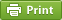Werner's Blog — Opinion, Analysis, Commentary
Structural decomposition analysis

A common task for empirical environmental economists and energy economists involves decomposing a change in emissions or energy use over time into its three main constituent parts:

• the scale effect that accounts for overall changes in economic activity such as GDP growth;
• the composition effect that accounts for the shift in sectoral activity such as moving from energy-intensive industries to service industries;
• the technique effect that accounts for changes in technology and emission abatement, caused either by innovation and technological progress, or by public policies.

Let us focus on emissions. Mathematically, the decomposition can be written down as $E_t= \sum_i E_{it} = \sum_i e_{it} q_{it} = \sum_i e_{it} s_{it} q_t$ where firm $$i$$ has total emissions $$E_{it}$$, emission intensity $$e_{it}$$ and output $$q_{it}$$. The latter can again be decomposed into firm $$i$$'s share $$s_{it}$$ in total output $$q_t$$. We are interested in figuring out the change over time, from base period $$b$$ to current period $$t$$. Specifically we want to decompose $E_t-E_b= \sum_i (e_{it}-e_{ib})x^T_i + (s_{it}-s_{ib})x^C_i + (q_t-q_b)x^S_i$ For this to work, we need to figure out weights $$x_i$$ for the technique, composition, and scale effect that solve the above equation exactly. The method for this is known as structural decomposition analysis. This methodology is a close cousin of index number theory. The goal is to find an "ideal" decomposition. The paper by Paul De Boer (2008) discusses the related concepts and points out the benefits of using the Montgomery decomposition. I find this method particularly intuitive, meeting the criteria for "ideal" decompositions, and thus would like to recommend its wider use. This blog summarizes some of the key points made in De Boer's paper.

The methodology is perhaps best explained with simple two-factor decomposition. Consider our example above for a single firm where there is only a technique and scale effect, but no composition effect. Then there are two elementary (polar) decompositions: $E_{it}-E_{ib}= (e_{it}-e_{ib})q_{it} +(q_{it}-q_{ib})e_{ib}$ $E_{it}-E_{ib}= (e_{it}-e_{ib})q_{ib} +(q_{it}-q_{ib})e_{it}$ These decompositions are considered "polar" because they are complete and have no residual term. They are also robust to zero values. Choosing one decomposition over the other would be problematic, so most researchers use the average of the two: $E_{it}-E_{ib}= (e_{it}-e_{ib})\left[\frac{q_{ib}+q_{it}}{2}\right] +(q_{it}-q_{ib})\left[\frac{e_{ib}+e_{it}}{2}\right]$ This type of decomposition becomes more awkward when there are more than two decomposition factors involved. Rather than having to average over two elementary decomposition, when there are $$k$$ factors, there are in fact $$k!$$ elementary decompositions. So for three factors there are 3!=6 elementary decompositions. Decompositions can still be made, but when there are many factors, it is easy to see that the methodology becomes unwieldy. The solution to the problem is the Montgomery decomposition that makes use of the logarithmic mean.

Unlike its three cousins, the arithmetic, geometric, and harmonic mean, the logarithmic mean is not widely known or used because it is confined to the domain of positive numbers. Yet it has some highly practical properties. In general, the logarithmic mean is larger than the geometric mean but smaller than the arithmetic mean. The logarithmic mean for two positive numbers $$x$$ and $$y$$ is defined as $L(x,y)\equiv\frac{x-y}{\ln(x)-\ln(y)} \quad\mathrm{and}\quad L(x,x)=x$ with the symmetry property $$L(x,y)=L(y,x)$$. Then it follows that $$x-y=\ln(x/y)L(x,y)$$. For a two-factor decomposition $$z_t=x_t y_t$$ and $$z_b=x_b y_b$$ it turns out that $$z_t-z_b=x_ty_t-x_by_b=L(z_t,z_b)\ln(z_t/z_b)$$, and we can make use of the fact that $$ln(z_t/z_b)=\ln(x_t/x_b)+\ln(y_t/y_b)$$. Then replace $$\ln(x_t/x_b)=(x_t-x_b)/L(x_t,x_b)$$ and do the same for $$y$$. Thus it holds that $$z_t-z_t=L(z_t,z_t)[ (x_t-x_t)/L(x_t,x_b)+(y_t-y_b)/L(y_t,y_b)]$$, and in turn we obtain the Montgomery decomposition $E_{it}-E_{ib}=\left[(e_{it}-e_{ib})\frac{L(E_{it},E_{ib})}{L(e_{it},e_{ib})} +(q_{it}-q_{ib})\frac{L(E_{it},E_{ib})}{L(q_{it},q_{ib})}\right]$ It is straight-forward to move from a two-factor to a three-factor or multi-factor decomposition. Going back to our starting point, the three decomposition factors in the second equation can be written as $x_i^T=\frac{L(E_{t},E_{b})}{L(e_{it},e_{ib})} \quad x_i^C=\frac{L(E_{t},E_{b})}{L(s_{it},s_{ib})} \quad x_i^S=\frac{L(E_{t},E_{b})}{L(q_{t},q_{b})}$

When calculating structural decompositions, we are mostly interested in percentage numbers. The overall percentage change can be calculated as $$100\%\cdot(E_t/E_b-1)$$. The technique effect, for example, could then be calculated as $\frac{100\%}{E_b}\cdot\sum_i (e_{it}-e_{ib})\frac{L(E_{t},E_{b})}{L(e_{it},e_{ib})}$

Using the Montgomery decomposition is really quite easy. For implementation, it is often useful to code the logarithmic mean as a function because it is rarely pre-defined. Here is a simple SAS code fragment.

options cmplib=work.funcs; proc fcmp outlib=work.funcs.MathFuncs; function logmean(x,y); if x<0 or y<0 then return(.); if x=0 or y=0 then return(0); if x=y then return(x); return((x-y)/(log(x)-log(y))); endsub; quit;

After calculating structural decompositions, the remaining problem involves displaying the information in an intuitive way. Overall changes are easily displayed in a bar diagram. However, decompositions can be both positive and negative, so using a simple stacked bar diagram will not suffice. In one of my next blogs I will introduce my favourite way of displaying structural decomposition output: a decomposition bar chart that is compact and intuitive.

Referenes and Sources:

Posted on Monday, January 8, 2018 at 09:00 — #EconometricsRecent Blog Entries

Topics

Months

Subscribe to RSS feed© 2023  Prof. Werner Antweiler, University of British Columbia.